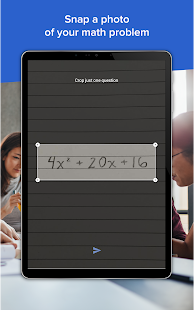Descriptions :

Mathway is the world's smartest math calculator for algebra, graphing, calculus, and more. Just point your camera and snap a photo or type your math homework question for step-by-step answers. With millions of users and billions of problems solved, Mathway is like a private tutor in the palm of your hand, providing help snapshot with homework anywhere. Mathway covers all levels of math, including: Basic Math / Pre-Algebra (arithmetic, integers, fractions, decimal numbers, roots, factors and more ...) Algebra (linear equations / inequalities, quadratic equations / inequalities, absolute equations / inequalities, systems of equations, logarithms, functions, matrices, graphs and more ...)

Required Android Versions : Marshmallow [6.0 - 6.0.1] - Nougat [7.0 – 7.1.1] - Oreo [8.0-8.1]